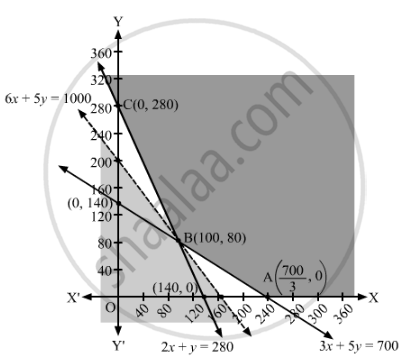# There Are Two Types of Fertilizers F1 and F2. F1 Consists of 10% Nitrogen and 6% Phosphoric Acid and ​F2 Consists of 5% Nitrogen and 10% Phosphoric Acid. - Mathematics

Sum

There are two types of fertilizers Fand F2. Fconsists of 10% nitrogen and 6% phosphoric acid and ​Fconsists of 5% nitrogen and 10% phosphoric acid. After testing the soil conditions, a farmer finds the she needs atleast 14 kg of nitrogen and 14 kg of phosphoric acid for her crop. If Fcosts ₹6/kg and Fcosts ₹5/kg, determine how much of each type of fertilizer should be used so that the nutrient requirements are met at minimum cost. What is the minimum cost?

#### Solution

Suppose x kg of fertilizer Fand and y kg of fertilizer F2 is used to meet the nutrient requirements.

Fconsists of 10% nitrogen and Fconsists of 5% nitrogen. But, the farmer needs atleast 14 kg of nitorgen for the crops.

∴ 10% of x kg + 5% of y kg ≥ 14 kg

$\Rightarrow \frac{x}{10} + \frac{y}{20} \geq 14$
$\Rightarrow 2x + y \geq 280$

Similarly, Fconsists of 6% phosphoric acid and Fconsists of 10% phosphoric acid. But, the farmer needs atleast 14 kg of phosphoric acid for the crops.

∴ 6% of x kg + 10% of y kg ≥ 14 kg

$\Rightarrow \frac{6x}{100} + \frac{10y}{100} \geq 14$
$\Rightarrow 3x + 5y \geq 700$
The cost of fertilizer Fis ₹6/kg and fertilizer Fis ₹5/kg, therefore, total cost of x kg of fertilizer Fand and y kg of fertilizer Fis ₹(6x + 5y).

Thus, the given linear programming problem is

Minimise Z = 6+ 5y

subject to the constraints

2x + ≥ 280

3x + 5≥ 700

x, y ≥ 0

The feasible region determined by the given constraints can be diagrammatically represented as,The coordinates of the corner points of the feasible region are $A\left( \frac{700}{3}, 0 \right), B ( 100,80 ) \text{ and C }( 0,280 )$ .

The value of the objective function at these points are given in the following table.

 Corner Point Z = 6x + 5y $\left( \frac{700}{3}, 0 \right)$ $6 \times \frac{700}{3} + 5 \times 0 = 1400$ (100, 80) 6 × 100 + 5 × 80 = 1000 → Minimum (0, 280) 6 × 0 + 5 × 280 = 1400
The smallest value of Z is 1000 which is obtained at x = 100, y = 80.

It can be seen that the open half-plane represented by 6x + 5y < 1000 has no common points with the feasible region.

So, the minimum value of Z is 1000.

Hence, 100 kg of fertilizer Fand and 80 kg of fertilizer F2 should be used so that the nutrient requirements are met at minimum cost. The minimum cost is ₹1,000.
Concept: Graphical Method of Solving Linear Programming Problems
Is there an error in this question or solution?

#### APPEARS IN

RD Sharma Class 12 Maths
Chapter 30 Linear programming
Exercise 30.4 | Q 47 | Page 56